# Modeling on Unbalanced Data: Caravan Insurance

## Overview¶

This notebook will look at the 2016 Kaggle Caravan Insurance Challenge. The data was collected to see with the following goal in mind:

Can you predict who would be interested in buying a caravan insurance policy and give an explanation why?

Several models will be used in attempting to answer this question:

• Bagging
• Boosting (2 variants)
• Random Forest

As we are working with unbalanced data, each model will have be run against a training dataset in 4 different states:

• Unbalanced (No modifications)
• Undersampled
• Oversampled
• SMOTE

#### Imports¶

In :
```#%matplotlib inline
import pandas as pd
import numpy as np
import matplotlib.pyplot as plt
import seaborn as sns

from sklearn.ensemble import BaggingClassifier, RandomForestClassifier, AdaBoostClassifier
from sklearn.model_selection import train_test_split
from sklearn.metrics import confusion_matrix, roc_auc_score, roc_curve, classification_report, f1_score
from lightgbm import LGBMClassifier

from imblearn.over_sampling import RandomOverSampler,SMOTE
from imblearn.under_sampling import RandomUnderSampler
from imblearn.metrics import classification_report_imbalanced

import itertools
import scipy.stats as ss
```
```C:\Users\Rygu\Anaconda3\envs\i4061\lib\site-packages\sklearn\externals\six.py:31: DeprecationWarning: The module is deprecated in version 0.21 and will be removed in version 0.23 since we've dropped support for Python 2.7. Please rely on the official version of six (https://pypi.org/project/six/).
"(https://pypi.org/project/six/).", DeprecationWarning)
```
In :
```RS = 404 # Random state
```

## Data¶

The dataset used is from the CoIL Challenge 2000 datamining competition. It may be obtained from: https://www.kaggle.com/uciml/caravan-insurance-challenge

It contains information on customers of an insurance company. The data consists of 86 variables and includes product usage data and socio-demographic data derived from zip area codes.

A description of each variable may be found at the link listed above.

Each number corresponds with a certain key, specific to each variable. There are 5 levels of keys, L0-L4 each key represents a different group range. As a sample:

``````L0 - Customer subtype (1-41)
1: High Income, expensive child
2: Very Important Provincials
3: High status seniors
...
L1 - average age keys (1-6):
1: 20-30 years
2: 30-40 years
3: 40-50 years
...
L2 - customer main type keys (1-10):
1: Successful hedonists
2: Driven Growers
3: Average Family
...
L3 - percentage keys (0-9):
0: 0%
1: 1 - 10%
2: 11 - 23%
3: 24 - 36%
...
L4 - total number keys (0-9):
0: 0
1: 1 - 49
2: 50 - 99
3: 100 - 199
...``````

The variable descriptions are quite important as it appears as though the variable names themselves are abbreviated in Dutch. One helpful pattern to notice is the letters that variables begin with:

• M - primary demographics?, no guess for abbreviation
• A - numbers, possibly for dutch word aantal
• P - percents, possibly for dutch word procent

Acknowledgements

P. van der Putten and M. van Someren (eds) . CoIL Challenge 2000: The Insurance Company Case. Published by Sentient Machine Research, Amsterdam. Also a Leiden Institute of Advanced Computer Science Technical Report 2000-09. June 22, 2000.

In :
```carav = pd.read_csv('data/caravan-insurance-challenge.csv')
```
Out:
ORIGIN MOSTYPE MAANTHUI MGEMOMV MGEMLEEF MOSHOOFD MGODRK MGODPR MGODOV MGODGE ... APERSONG AGEZONG AWAOREG ABRAND AZEILPL APLEZIER AFIETS AINBOED ABYSTAND CARAVAN
0 train 33 1 3 2 8 0 5 1 3 ... 0 0 0 1 0 0 0 0 0 0
1 train 37 1 2 2 8 1 4 1 4 ... 0 0 0 1 0 0 0 0 0 0
2 train 37 1 2 2 8 0 4 2 4 ... 0 0 0 1 0 0 0 0 0 0
3 train 9 1 3 3 3 2 3 2 4 ... 0 0 0 1 0 0 0 0 0 0
4 train 40 1 4 2 10 1 4 1 4 ... 0 0 0 1 0 0 0 0 0 0

5 rows × 87 columns

Since the competition has ended, all the data has been made available as one large concatenated dataset. Luckily, in doing so they've also added an additional column "ORIGIN" which indicated where the data was originally from, so we can simulate what the competition was initially like.

In :
```carav.ORIGIN.value_counts()
```
Out:
```train    5822
test     4000
Name: ORIGIN, dtype: int64```

## Exploratory Data Analysis¶

No NA values, all variables are type of int64. The data is peculiar in that every numeric value stands for an attribute of a person. Even variables that could be continuous, such as income, have been binned. In this sense, this dataset is entirely comprised of Categorical and Ordinal values. Other than potential collinearity between percentage and range values, the data is mostly clean.

For the EDA portion, we will cheat a bit and use knowledge from the combined dataset to get a better picture of the data. During the actual competition, contestants would not have had access to the `CARAVAN` variable where `ORIGIN` = `test`

In :
```carav.info()
```
```<class 'pandas.core.frame.DataFrame'>
RangeIndex: 9822 entries, 0 to 9821
Data columns (total 87 columns):
ORIGIN      9822 non-null object
MOSTYPE     9822 non-null int64
MAANTHUI    9822 non-null int64
MGEMOMV     9822 non-null int64
MGEMLEEF    9822 non-null int64
MOSHOOFD    9822 non-null int64
MGODRK      9822 non-null int64
MGODPR      9822 non-null int64
MGODOV      9822 non-null int64
MGODGE      9822 non-null int64
MRELGE      9822 non-null int64
MRELSA      9822 non-null int64
MRELOV      9822 non-null int64
MFALLEEN    9822 non-null int64
MFGEKIND    9822 non-null int64
MFWEKIND    9822 non-null int64
MOPLHOOG    9822 non-null int64
MOPLMIDD    9822 non-null int64
MOPLLAAG    9822 non-null int64
MBERHOOG    9822 non-null int64
MBERZELF    9822 non-null int64
MBERBOER    9822 non-null int64
MBERMIDD    9822 non-null int64
MBERARBG    9822 non-null int64
MBERARBO    9822 non-null int64
MSKA        9822 non-null int64
MSKB1       9822 non-null int64
MSKB2       9822 non-null int64
MSKC        9822 non-null int64
MSKD        9822 non-null int64
MHHUUR      9822 non-null int64
MHKOOP      9822 non-null int64
MAUT1       9822 non-null int64
MAUT2       9822 non-null int64
MAUT0       9822 non-null int64
MZFONDS     9822 non-null int64
MZPART      9822 non-null int64
MINKM30     9822 non-null int64
MINK3045    9822 non-null int64
MINK4575    9822 non-null int64
MINK7512    9822 non-null int64
MINK123M    9822 non-null int64
MINKGEM     9822 non-null int64
MKOOPKLA    9822 non-null int64
PWAPART     9822 non-null int64
PWABEDR     9822 non-null int64
PWALAND     9822 non-null int64
PPERSAUT    9822 non-null int64
PBESAUT     9822 non-null int64
PMOTSCO     9822 non-null int64
PVRAAUT     9822 non-null int64
PAANHANG    9822 non-null int64
PTRACTOR    9822 non-null int64
PWERKT      9822 non-null int64
PBROM       9822 non-null int64
PLEVEN      9822 non-null int64
PPERSONG    9822 non-null int64
PGEZONG     9822 non-null int64
PWAOREG     9822 non-null int64
PBRAND      9822 non-null int64
PZEILPL     9822 non-null int64
PPLEZIER    9822 non-null int64
PFIETS      9822 non-null int64
PINBOED     9822 non-null int64
PBYSTAND    9822 non-null int64
AWAPART     9822 non-null int64
AWABEDR     9822 non-null int64
AWALAND     9822 non-null int64
APERSAUT    9822 non-null int64
ABESAUT     9822 non-null int64
AMOTSCO     9822 non-null int64
AVRAAUT     9822 non-null int64
AAANHANG    9822 non-null int64
ATRACTOR    9822 non-null int64
AWERKT      9822 non-null int64
ABROM       9822 non-null int64
ALEVEN      9822 non-null int64
APERSONG    9822 non-null int64
AGEZONG     9822 non-null int64
AWAOREG     9822 non-null int64
ABRAND      9822 non-null int64
AZEILPL     9822 non-null int64
APLEZIER    9822 non-null int64
AFIETS      9822 non-null int64
AINBOED     9822 non-null int64
ABYSTAND    9822 non-null int64
CARAVAN     9822 non-null int64
dtypes: int64(86), object(1)
memory usage: 6.5+ MB
```

One nice feature of this dataset is that every feature is already encoded as an integer representation, saving us the conversion work. The downside of this is that, without reading the dataset description, it isn't easily interpretable, particularly true for those who do not understand Dutch, as well.

In :
```carav.CARAVAN.value_counts()
```
Out:
```0    9236
1     586
Name: CARAVAN, dtype: int64```

We can see that we are dealing with a very imbalanced dataset, if this is not factored in during modeling, any predictions will be wildly biased toward non-policy holders.

In :
```plt.subplots(figsize=(8,6))
sns.heatmap(carav.drop(columns=['ORIGIN']).corr());
```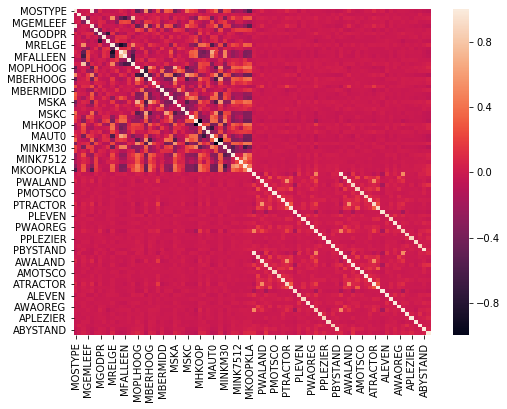A correlation plot reveals some rather interesting pattens in the data. There is a clear divide between the two groupings listed in the description file with keys L3 and L4

In :
```fig, axes = plt.subplots(1,2, figsize=(16,6))

sns.heatmap(carav.drop(columns=['ORIGIN']).iloc[:,:43].corr(), vmin=-1, vmax=1, cmap='coolwarm',ax=axes)
sns.heatmap(carav.drop(columns=['ORIGIN']).iloc[:,43:].corr(), vmin=-1, vmax=1, cmap='coolwarm',ax=axes)
axes.set_title("L3 keys: Upper-left Corrplot")
axes.set_title("L4 keys: Bottom-right Corrplot");
```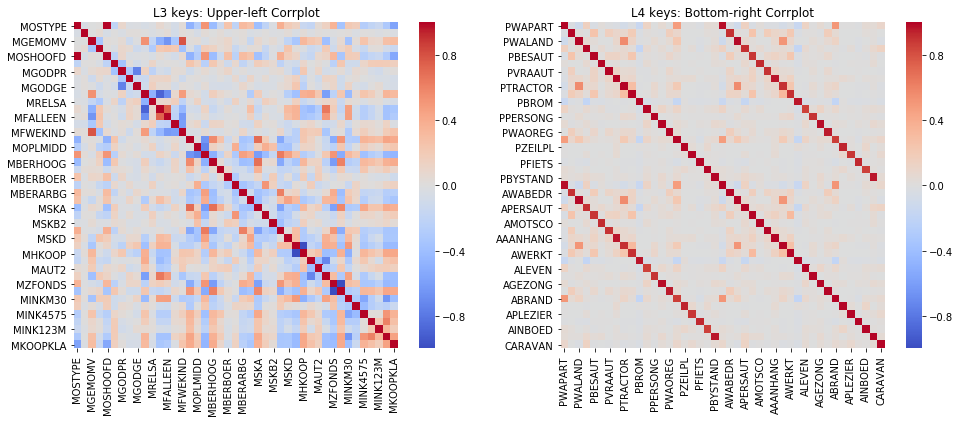After zooming in a bit, the L4 keys plot (right) shows how variables starting with `P` each have a corresponding variable starting with `A` this means that having both in our data will likely provide little value.

In :
```# To see a numeric representation of the heatmaps
# carav.loc[:,(carav.columns.str.startswith('P') | carav.columns.str.startswith('A'))].corr()
```
In :
```# Drop percentage representations, keep raw number range
carav_np = carav.drop(columns=carav.loc[:,(carav.columns.str.startswith('P'))]).copy()
carav_np.to_feather('data/reduced_cmbd.df')
```

Note: to be test our theory, multiple models would need to be tested on those with and without the percentage representation.

## Models¶

4 Models will be used in total: `BaggingClassifier`, `RandomForestClassifier`, `AdaBoostClassifier` from sklearn and Microsoft's `lightgbm`

#### Helper functions¶

In :
```def plot_confusion_matrix(y_true, y_pred, classes,
normalize=False, cf_report=False,
title='Confusion matrix', ax=None, cmap=plt.cm.Blues, cbar=False):
"""
This function prints and plots the confusion matrix.
Normalization can be applied by setting `normalize=True`.
"""
cm = confusion_matrix(y_true, y_pred)
if normalize:
cm = cm.astype('float') / cm.sum(axis=1)[:, np.newaxis]

if cf_report:
print(classification_report(y_true,y_pred))

fig, ax = (plt.gcf(), ax) if ax is not None else plt.subplots(1,1)

im = ax.imshow(cm, interpolation='nearest', cmap=cmap)
ax.set_title(title)

if cbar:
fig.colorbar(im, ax=ax, fraction=0.046, pad=0.04) # "Magic" numbers (https://stackoverflow.com/a/26720422/10939610)

tick_marks = np.arange(len(classes))
ax.set_xticks(tick_marks)
ax.set_xticklabels(classes, rotation=45)
ax.set_yticks(tick_marks)
ax.set_yticklabels(classes)

fmt = '.2f' if normalize else 'd'
thresh = cm.max() / 2.
for i, j in itertools.product(range(cm.shape), range(cm.shape)):
ax.text(j, i, format(cm[i, j], fmt),
horizontalalignment="center",
color="white" if cm[i, j] > thresh else "black")

fig.tight_layout()
ax.set_ylabel('True label')
ax.set_xlabel('Predicted label')
```
In :
```def plot_roc(y_true, y_pred, ax=None):
"""Plot ROC curve"""
false_positive_rate, true_positive_rate, threshold = roc_curve(y_true, y_pred)
roc_score = roc_auc_score(y_true,y_pred)

fig, ax = (plt.gcf(), ax) if ax is not None else plt.subplots(1,1)

ax.plot(false_positive_rate, true_positive_rate)
ax.plot([0, 1], ls="--")
ax.plot([0, 0], [1, 0] , c=".7"), plt.plot([1, 1] , c=".7")
ax.annotate('ROC: {:.5f}'.format(roc_score), [0.75,0.05])
ax.set_ylabel("True Positive Rate")
ax.set_xlabel("False Positive Rate")
fig.tight_layout()
return roc_score
```
In :
```def feat_imps(model, X_train, plot=False, n=None):
""" Dataframe containing each feature with its corresponding importance in the given model

Args
----
model : model, classifier that supports .feature_importances_ (RandomForest, AdaBoost, ect..)
X_train : array like, training data object
plot : boolean, if True, plots the data in the form of a bargraph
n : int, only applicable if plot=True, number of features to plot, (default=15)

Returns
-------
pandas DataFrame : columns = feature name, importance
"""

fi_df = pd.DataFrame({'feature':X_train.columns,
'importance':model.feature_importances_}
).sort_values(by='importance', ascending=False)
if plot:
fi_df[:(n if n is not None else 15)].plot.bar(x='feature',y='importance')
else:
return fi_df
```
In :
```def plot_cmroc(y_true, y_pred, classes=[0,1], normalize=True, cf_report=False):
"""Convenience function to plot confusion matrix and ROC curve """
fig,axes = plt.subplots(1,2, figsize=(9,4))
plot_confusion_matrix(y_true, y_pred, classes=classes, normalize=normalize, cf_report=cf_report, ax=axes)
roc_score = plot_roc(y_true, y_pred, ax=axes)
fig.tight_layout()
plt.show()
return roc_score
```

A few helper functions that will be used extensively during modeling

In :
```train_df = carav.query("ORIGIN=='train'").iloc[:,1:].copy()
test_df = carav.query("ORIGIN=='test'").iloc[:,1:].copy()
```

The test data will be treated as a holdout test set, so we will split `train_df` into a training validation set. This more closely resembles how the actual competition would have been set up.

In :
```X, y = train_df.drop(columns='CARAVAN'), train_df.CARAVAN
X_train, X_val, y_train, y_val = train_test_split(X, y, test_size=0.20, random_state=RS)
```

To address the issue with imbalanced data, we will compare three approaches for each model used:

• Random Over Sampling - attempts to balance the data by randomly selecting from the minority class, in this case, those who did purchase a caravan insurance policy.

• Random Under Sampling - balances data by randomly under under selecting from the majority class, those who did not purchase caravan insurance.

• SMOTE - Synthetic Minority Over-sampling Technique, constructs new synthetic data by sampling neighboring points. Balancing happens through both oversampling the minority and undersampling the major class

In :
```ros = RandomOverSampler(random_state=RS)
rus = RandomUnderSampler(random_state=RS)
smt = SMOTE(random_state=RS, n_jobs=-1)
```
In :
```X_under, y_under = rus.fit_sample(X_train,y_train)
X_over, y_over = ros.fit_sample(X_train,y_train)
X_smote, y_smote = smt.fit_sample(X_train,y_train)
```
In :
```pd.DataFrame([*map(lambda x: ss.describe(x)._asdict(),[y_train,y_under,y_over,y_smote])],
index=['Unbalanced','Undersample','Oversample','SMOTE'])
```
Out:
nobs minmax mean variance skewness kurtosis
Unbalanced 4657 (0, 1) 0.059695 0.056144 3.716892 11.815283
Undersample 556 (0, 1) 0.500000 0.250450 0.000000 -2.000000
Oversample 8758 (0, 1) 0.500000 0.250029 0.000000 -2.000000
SMOTE 8758 (0, 1) 0.500000 0.250029 0.000000 -2.000000

Without doing any sort of resampling, the mean was ~0.06 with a heavy skew. Each method of resampling has shifted the mean to 0.5 and eliminated the skewness, each using a different method to achieve this.

In :
```# Define our baseline models
bc = BaggingClassifier(n_estimators=53, random_state=RS, n_jobs=-1)
rfc = RandomForestClassifier(n_estimators=53, random_state=RS, n_jobs=-1)
lgbm = LGBMClassifier(n_estimators=53, random_state=RS, n_jobs=-1)
```

### Unbalanced Data¶

#### Bagging¶

In :
```bc_unbal = plot_cmroc(y_val, bc.fit(X_train, y_train).predict(X_val))
```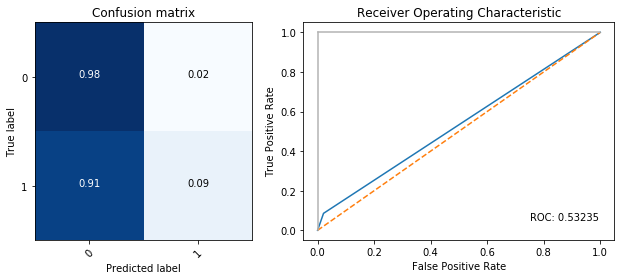In :
```ada_unbal = plot_cmroc(y_val, ada.fit(X_train, y_train).predict(X_val))
```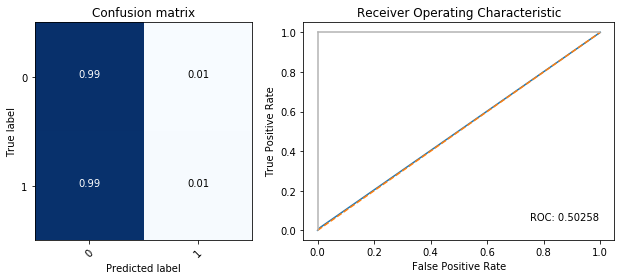#### Random Forest¶

In :
```rfc_unbal = plot_cmroc(y_val, rfc.fit(X_train, y_train).predict(X_val))
```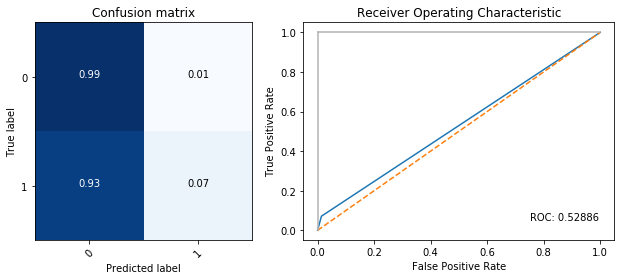#### Boosting (LGBM)¶

In :
```lgbm_unbal = plot_cmroc(y_val, lgbm.fit(X_train, y_train).predict(X_val))
```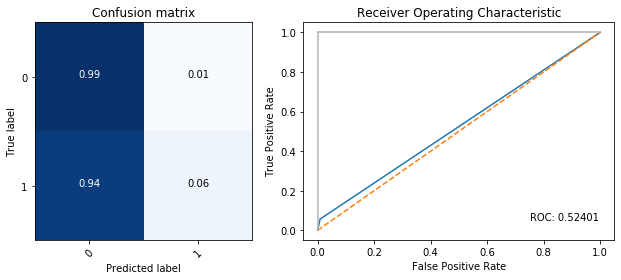#### Unbalanced Evaluation¶

In :
```unbal_scores = [bc_unbal, ada_unbal, rfc_unbal, lgbm_unbal]

for model, score in zip(models, unbal_scores):
print('{:25s}: {:.5f}'.format(model.__class__.__name__, score))
```
```BaggingClassifier        : 0.53235
RandomForestClassifier   : 0.52886
LGBMClassifier           : 0.52401
```

Poor performance across all models when using the unbalanced dataset. AdaBoost was no better than random guessing and the best model, the BaggingClassifier, was barely beyond that.

### Undersampling¶

#### Bagging¶

In :
```bc_under = plot_cmroc(y_val, bc.fit(X_under, y_under).predict(X_val))
```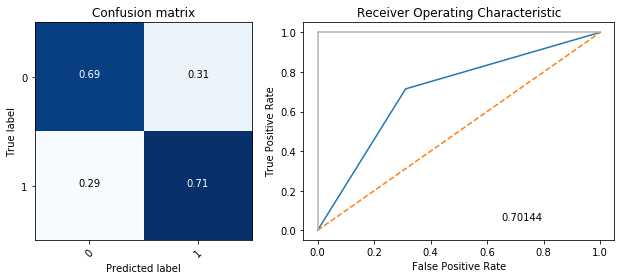In :
```ada_under = plot_cmroc(y_val, ada.fit(X_under, y_under).predict(X_val))
```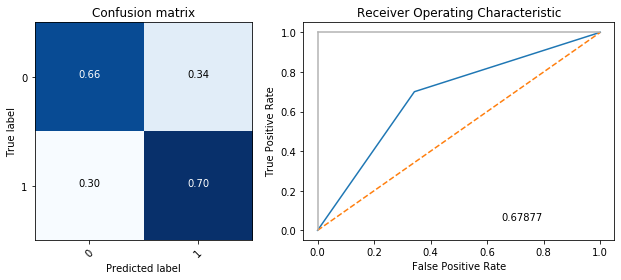#### Random Forest¶

In :
```rfc_under = plot_cmroc(y_val, rfc.fit(X_under, y_under).predict(X_val))
```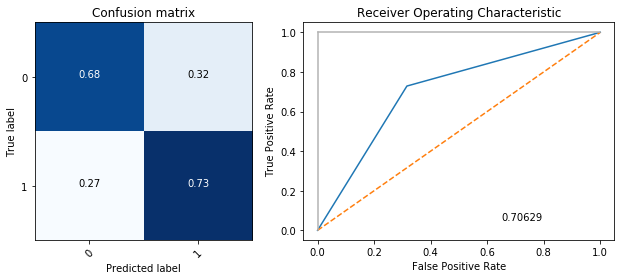#### Boosting (LGBM)¶

In :
```lgbm_under = plot_cmroc(y_val, lgbm.fit(X_under, y_under).predict(X_val))
```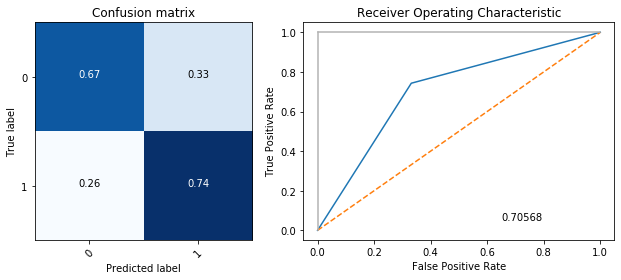#### Undersampling Evaluation¶

In :
```under_scores = [bc_under, ada_under, rfc_under, lgbm_under]

for model, score in zip(models, under_scores):
print('{:25s}: {:.5f}'.format(model.__class__.__name__, score))
```
```BaggingClassifier        : 0.70144
RandomForestClassifier   : 0.70629
LGBMClassifier           : 0.70568
```

Nearly a 20% increase in ROC score was seen across the board using the undersampling method.

### Oversampling¶

#### Bagging¶

In :
```bc_over = plot_cmroc(y_val, bc.fit(X_over, y_over).predict(X_val))
```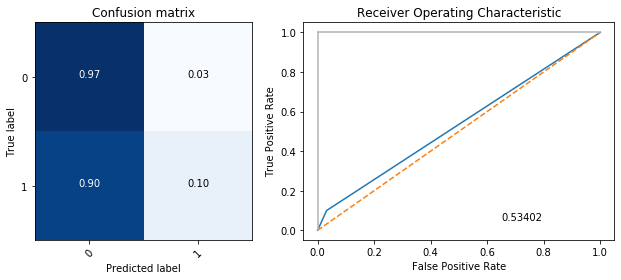In :
```ada_over = plot_cmroc(y_val, ada.fit(X_over, y_over).predict(X_val))
```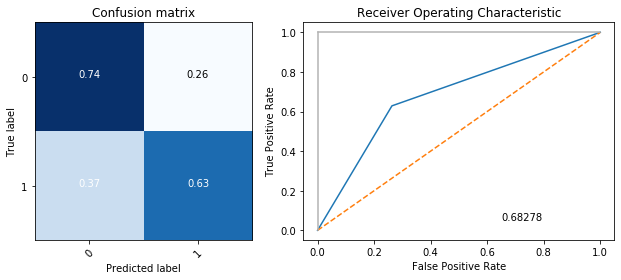#### Random Forest¶

In :
```rfc_over = plot_cmroc(y_val, rfc.fit(X_over, y_over).predict(X_val))
```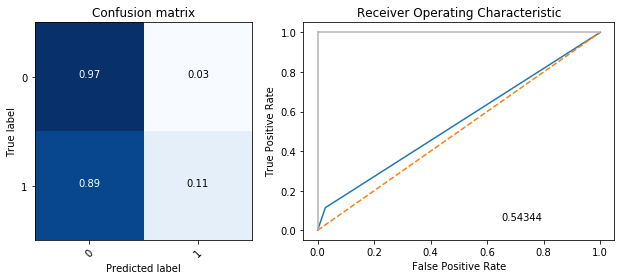#### Boosting (LGBM)¶

In :
```lgbm_over = plot_cmroc(y_val, lgbm.fit(X_over, y_over).predict(X_val))
```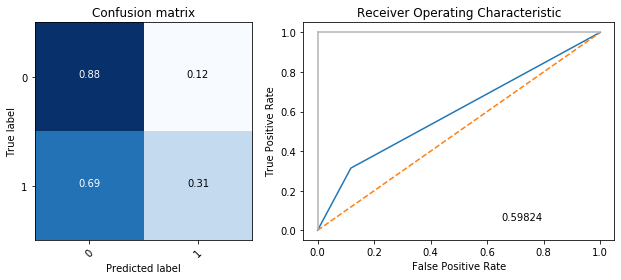#### Oversampling Evaluation¶

In :
```over_scores = [bc_over, ada_over, rfc_over, lgbm_over]

for model, score in zip(models, over_scores):
print('{:25s}: {:.5f}'.format(model.__class__.__name__, score))
```
```BaggingClassifier        : 0.53402
RandomForestClassifier   : 0.54344
LGBMClassifier           : 0.59824
```

In contrast with the unbalanced dataset, with the over sampled data, AdaBoost greatly out performed the other models with this data augmentation method. It still fell shy of the ROC score achieved with undersampling.

### SMOTE¶

#### Bagging¶

In :
```bc_smote = plot_cmroc(y_val, bc.fit(X_smote, y_smote).predict(X_val))
```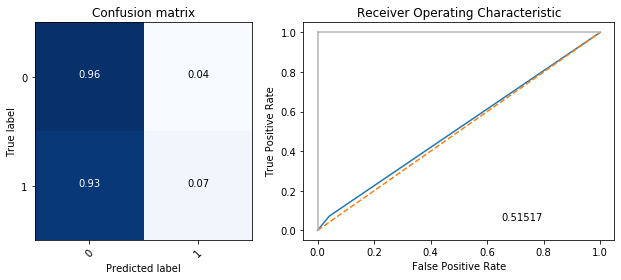In :
```ada_smote = plot_cmroc(y_val, ada.fit(X_smote, y_smote).predict(X_val))
```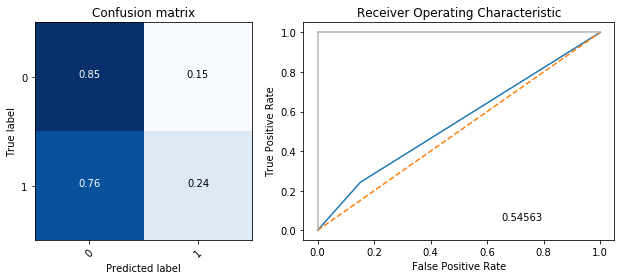#### Random Forest¶

In :
```rfc_smote = plot_cmroc(y_val, rfc.fit(X_smote, y_smote).predict(X_val))
```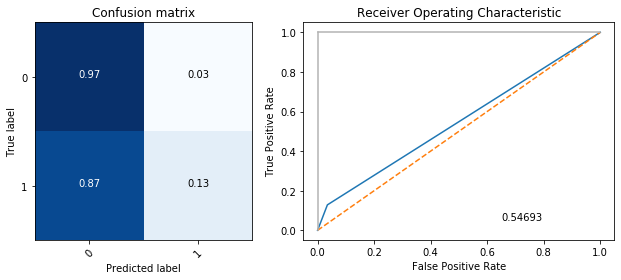#### Boosting (LGBM)¶

In :
```lgbm_smote = plot_cmroc(y_val, lgbm.fit(X_smote, y_smote).predict(X_val))
```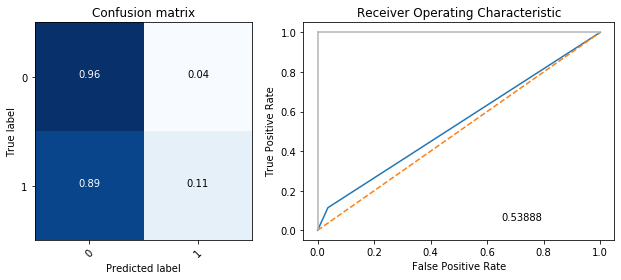#### SMOTE Evaluation¶

In :
```smote_scores = [bc_smote, ada_smote, rfc_smote, lgbm_smote]

for model, score in zip(models, smote_scores):
print('{:25s}: {:.5f}'.format(model.__class__.__name__, score))
```
```BaggingClassifier        : 0.51517
RandomForestClassifier   : 0.54693
LGBMClassifier           : 0.53888
```

### Tweaking the Best¶

For all of the classifiers, Random under sampling was the most successful method of rebalancing the dataset. With the exception of AdaBoost, the other methods barely outperformed random guessing.

Let's evaluate the best from each group against the holdout test dataset to see what we would have scored had this contest been live.

In :
```X_test, y_test = test_df.iloc[:,:-1], test_df.iloc[:,-1]
```
In :
```bc = BaggingClassifier(n_estimators=53,n_jobs=-1)
rfc = RandomForestClassifier(n_estimators=53,n_jobs=-1,random_state=RS)
lgbm = LGBMClassifier(n_estimators=53,random_state=RS)
```
In :
```for model in models:
model.fit(X_under,y_under)
tpreds = model.predict(X_test)
print('{:25s}: {:.5f}'.format(model.__class__.__name__,roc_auc_score(y_test,tpreds)))
```
```BaggingClassifier        : 0.64729
RandomForestClassifier   : 0.65894
LGBMClassifier           : 0.63902
```

So, if this contest happened to evaluated on Area Under ROC, the best model we could have submitted would have been the Random Forest Classifier, with a score of 0.65.

A bit better of a score could likely be achieved through ensembling these models as well, but there are many other tweaks that should tried before taking that route.

In :
```from sklearn.model_selection import GridSearchCV

param_grid = {
'learning_rate': [0.01, 0.05, 0.1, 1],
'n_estimators': [20,40,60,80,100],
'num_leaves':[3,7,17,31],
'max_bin': [4,8,16,32,64],
'min_child_samples':[3,5,10,20,30],
}
```

LGBM on Training/Validation set (Approx. 2-3 Minutes to run)

In :
```# eval_metric='auc' Remove random state to expedite search
# Setting iid = False will minimize the mean loss across the folds rather than for each fold individually
lgbm_gs = GridSearchCV(LGBMClassifier(), param_grid, n_jobs=-1, scoring='roc_auc', verbose=2, iid=False, cv=5)

lgbm_gs.fit(X_under, y_under)

print('Best parameters:', lgbm_gs.best_params_)
```
```Fitting 5 folds for each of 2000 candidates, totalling 10000 fits
```
```[Parallel(n_jobs=-1)]: Using backend LokyBackend with 4 concurrent workers.
[Parallel(n_jobs=-1)]: Done  68 tasks      | elapsed:    1.6s
[Parallel(n_jobs=-1)]: Done 1278 tasks      | elapsed:   16.6s
[Parallel(n_jobs=-1)]: Done 3308 tasks      | elapsed:   42.6s
[Parallel(n_jobs=-1)]: Done 6138 tasks      | elapsed:  1.3min
[Parallel(n_jobs=-1)]: Done 9788 tasks      | elapsed:  2.1min
```
```Best parameters: {'learning_rate': 0.1, 'max_bin': 16, 'min_child_samples': 3, 'n_estimators': 40, 'num_leaves': 7}
```
```[Parallel(n_jobs=-1)]: Done 10000 out of 10000 | elapsed:  2.1min finished
```
In :
```plot_cmroc(y_val, lgbm_gs.predict(X_val))
```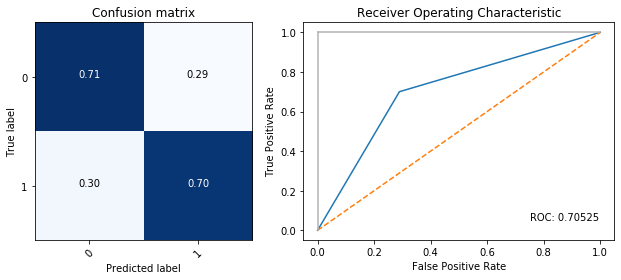Out:
`0.7052511415525115`
In :
```plot_cmroc(y_test, lgbm_gs.predict(X_test))
```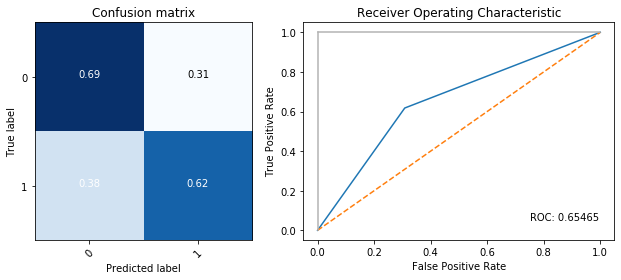Out:
`0.6546502173437159`

Random Forest (Approx. 1-2 minutes to run)

In :
```param_grid_rf = {
'n_estimators': [40,60,100,128,256],
'min_samples_leaf':[3,7,17,31],
'max_leaf_nodes': [4,8,16,32,64],
'min_samples_split':[3,5,10,20,30],
}
```
In :
```rfc_gs = GridSearchCV(RandomForestClassifier(), param_grid_rf, n_jobs=-1, scoring='roc_auc', verbose=2, iid=False, cv=5)

rfc_gs.fit(X_under, y_under)

print('Best parameters:', rfc_gs.best_params_)
```
```Fitting 5 folds for each of 500 candidates, totalling 2500 fits
```
```[Parallel(n_jobs=-1)]: Using backend LokyBackend with 4 concurrent workers.
[Parallel(n_jobs=-1)]: Done  33 tasks      | elapsed:    3.0s
[Parallel(n_jobs=-1)]: Done 154 tasks      | elapsed:    7.6s
[Parallel(n_jobs=-1)]: Done 357 tasks      | elapsed:   15.3s
[Parallel(n_jobs=-1)]: Done 640 tasks      | elapsed:   26.1s
[Parallel(n_jobs=-1)]: Done 1005 tasks      | elapsed:   40.3s
[Parallel(n_jobs=-1)]: Done 1450 tasks      | elapsed:   59.7s
[Parallel(n_jobs=-1)]: Done 1977 tasks      | elapsed:  1.4min
```
```Best parameters: {'max_leaf_nodes': 32, 'min_samples_leaf': 7, 'min_samples_split': 20, 'n_estimators': 60}
```
```[Parallel(n_jobs=-1)]: Done 2500 out of 2500 | elapsed:  1.8min finished
```
In :
```plot_cmroc(y_val, rfc_gs.predict(X_val))
```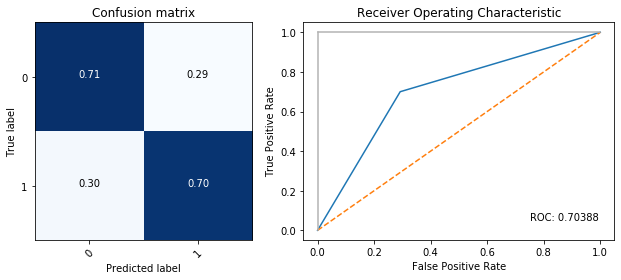Out:
`0.7038812785388128`
In :
```plot_cmroc(y_test, rfc_gs.predict(X_test))
```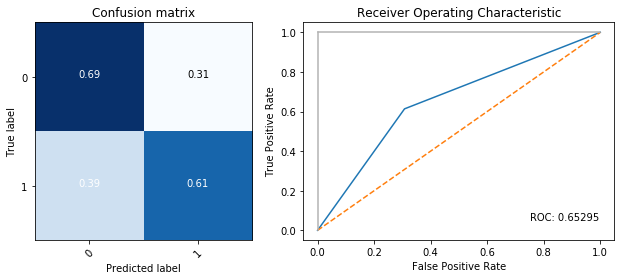Out:
`0.6529481010905159`

Both models saw no improvement in ROC scores compared to the non-grid search approaches. Neither the training evaluation, nor the test evaluation. Let's see how they do with the original, non-resampled data.

In :
```lgbm_gs_ub = GridSearchCV(LGBMClassifier(), param_grid, n_jobs=-1, scoring='roc_auc', verbose=1, iid=False, cv=5)
lgbm_gs_ub.fit(X_train, y_train)
print('Best parameters:', lgbm_gs_ub.best_params_)
```
```Fitting 5 folds for each of 2000 candidates, totalling 10000 fits
```
```[Parallel(n_jobs=-1)]: Using backend LokyBackend with 4 concurrent workers.
[Parallel(n_jobs=-1)]: Done  42 tasks      | elapsed:    4.3s
[Parallel(n_jobs=-1)]: Done 192 tasks      | elapsed:   13.4s
[Parallel(n_jobs=-1)]: Done 442 tasks      | elapsed:   28.4s
[Parallel(n_jobs=-1)]: Done 792 tasks      | elapsed:   49.9s
[Parallel(n_jobs=-1)]: Done 1242 tasks      | elapsed:  1.3min
[Parallel(n_jobs=-1)]: Done 1792 tasks      | elapsed:  1.9min
[Parallel(n_jobs=-1)]: Done 2442 tasks      | elapsed:  2.5min
[Parallel(n_jobs=-1)]: Done 3192 tasks      | elapsed:  3.3min
[Parallel(n_jobs=-1)]: Done 4042 tasks      | elapsed:  4.1min
[Parallel(n_jobs=-1)]: Done 4992 tasks      | elapsed:  5.0min
[Parallel(n_jobs=-1)]: Done 6042 tasks      | elapsed:  6.0min
[Parallel(n_jobs=-1)]: Done 7192 tasks      | elapsed:  7.1min
[Parallel(n_jobs=-1)]: Done 8442 tasks      | elapsed:  8.2min
[Parallel(n_jobs=-1)]: Done 9792 tasks      | elapsed:  9.4min
```
```Best parameters: {'learning_rate': 0.1, 'max_bin': 8, 'min_child_samples': 10, 'n_estimators': 80, 'num_leaves': 3}
```
```[Parallel(n_jobs=-1)]: Done 10000 out of 10000 | elapsed:  9.5min finished
```
In :
```plot_cmroc(y_val, lgbm_gs_ub.predict(X_val))
```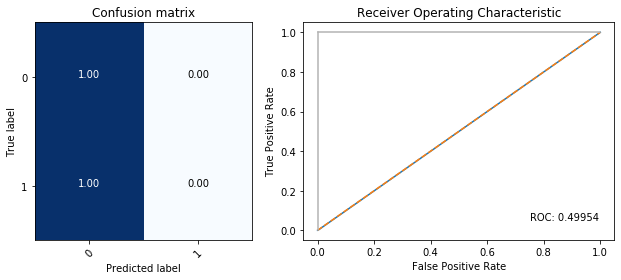Out:
`0.4995433789954338`
In :
```plot_cmroc(y_test, lgbm_gs_ub.predict(X_test))
```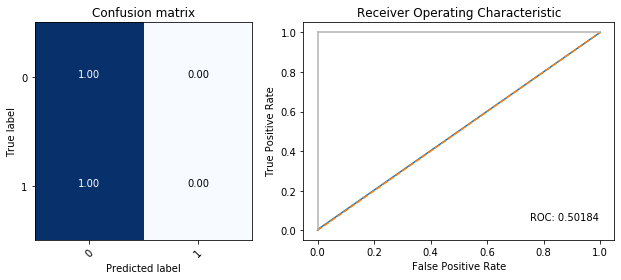Out:
`0.5018350242808447`
In :
```# RF
```
In :
```rfc_gs_ub = GridSearchCV(RandomForestClassifier(), param_grid_rf, n_jobs=-1, scoring='roc_auc', verbose=2, iid=False, cv=5)
rfc_gs_ub.fit(X_train, y_train)

print('Best parameters:', rfc_gs_ub.best_params_)
```
```Fitting 5 folds for each of 500 candidates, totalling 2500 fits
```
```[Parallel(n_jobs=-1)]: Using backend LokyBackend with 4 concurrent workers.
[Parallel(n_jobs=-1)]: Done  33 tasks      | elapsed:    2.6s
[Parallel(n_jobs=-1)]: Done 154 tasks      | elapsed:   13.3s
[Parallel(n_jobs=-1)]: Done 357 tasks      | elapsed:   30.8s
[Parallel(n_jobs=-1)]: Done 640 tasks      | elapsed:   56.7s
[Parallel(n_jobs=-1)]: Done 1005 tasks      | elapsed:  1.5min
[Parallel(n_jobs=-1)]: Done 1450 tasks      | elapsed:  2.4min
[Parallel(n_jobs=-1)]: Done 1977 tasks      | elapsed:  3.6min
[Parallel(n_jobs=-1)]: Done 2500 out of 2500 | elapsed:  4.9min finished
```
```Best parameters: {'max_leaf_nodes': 4, 'min_samples_leaf': 7, 'min_samples_split': 30, 'n_estimators': 100}
```
In :
```plot_cmroc(y_val, rfc_gs_ub.predict(X_val))
```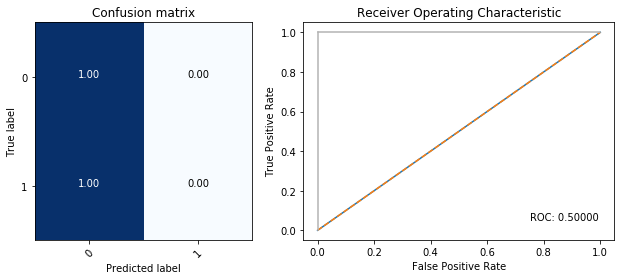Out:
`0.5`
In :
```plot_cmroc(y_test, rfc_gs_ub.predict(X_test))
```Out:
`0.5`

## Conclusions¶

In this notebook, we explored the CoIL Challenge 2000 datamining competition dataset. 4 Different models were used:
A BaggingClassifer, AdaBoost, Random Forrest, and LightGBM.

For each of these models, we 4 variants of the same training dataset:
Unaltered, Undersampled, Oversampled, and SMOTE.

We determined that without altering the data, the ROC score is no better than randomly guessing, Oversampling and SMOTE performed slightly better, but Undersampling was clearly the best approach.

After testing each model with the data modifications, a brute force method of Hyperparameter tuning was attempted via GridSearch followed by an automated means of feature selection. Neither of these methods yielded substantially better results for the compute time they required.

The highest end ROC score we were able to achieve in the synthetic competition environment was 0.66 with a overtuned Random Forest. The highest local test score was 0.784, showing that the model was clearly beginning to overfit the data.

At this time, I am unable to answer the question of:

Who is interested in buying Caravan Insurance and why?

with any degree of certainty. The winner of the 2000 challenge determined the strongest indicators variables were number of car policies, buying power, and various other policies held.

Future work: Countless parameters have been left untweaked, each model could have it's own grid search with each hyperparameter explored. As mentioned earlier, there was an issue with collinearity between percentage variables and number variables, this should be explored further. There is a great deal of EDA left undone, deeper relationships between variables should be investigated through interactions and transformations. Additionally, since this dataset is comprised largely of categorical variables, ordinal or otherwise, CatBoost might be a pragmatic choice for modeling, even attempting to use a Neural Network may yield interesting results.

Tags:

Categories:

Updated: